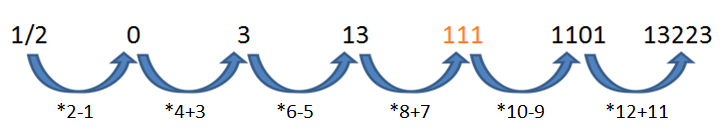We do not support landscape mode yet.
Please go back to portrait mode for the best experience
Question of The Day18-11-2019

Which number will replace the question mark (?) in the following question?

1/2, 0, 3, 13, ?, 1101, 13223

Correct Answer : b ) 111

Explanation :

Considering the given series

$$1 \over 2$$, 0, 3, 13, X, 1101, 13223

To obtain the next number from the previous number, the following logic will be applied:

• The number is first multiplied by twice the position at which the number is.
• Then the series of first 6 odd natural numbers is subtracted or added from the number.
• If the number thus obtained is at the odd position, then a number from series of odd natural numbers is subtracted from it.
• If the number thus obtained is at even position, then a number from series of odd natural numbers is added to it.

The logic in the above series can be explained asThus, X = 111

Hence, (b) is the correct answer.

Such type of question is asked in various government exams like SSC CGL, SSC MTS, SSC CPO, SSC CHSL, RRB JE, RRB NTPC, RRB GROUP D, RRB OFFICER SCALE-I, IBPS PO, IBPS SO, RRB Office Assistant, IBPS Clerk, RBI Assistant, IBPS RRB OFFICER SCALE 2&3, etc. Read Daily Current Affairs, Banking Affairs and other study material at PendulumEdu.